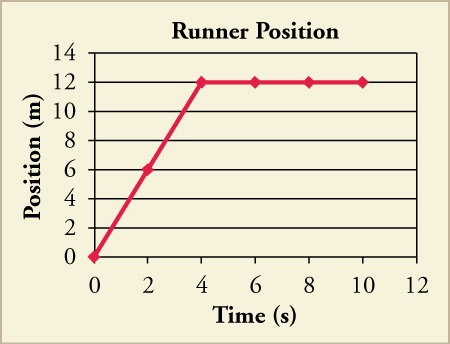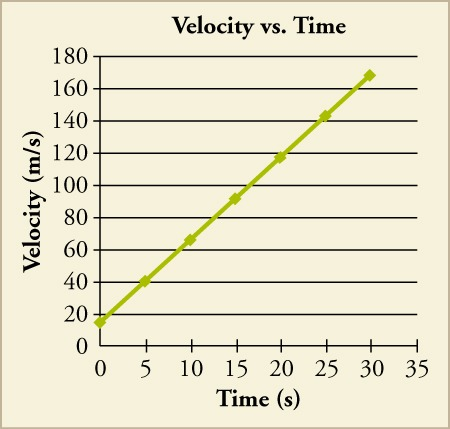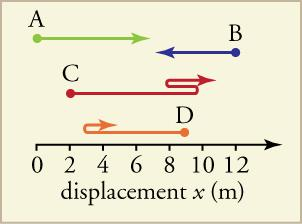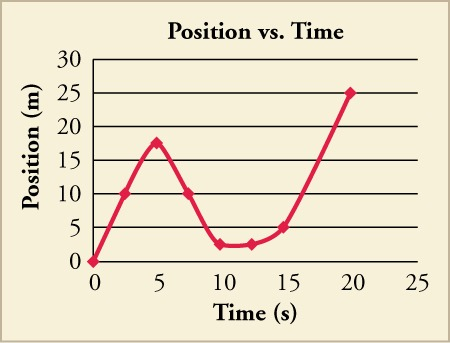Sections
Test Prep

# Test Prep

### Multiple Choice

#### 2.1Relative Motion, Distance, and Displacement

1.
Why should you specify a reference frame when describing motion?
1. a description of motion depends on the reference frame
2. motion appears the same in all reference frames
3. reference frames affect the motion of an object
4. you can see motion better from certain reference frames
2.
Which of the following is true for the displacement of an object?
1. It is always equal to the distance the object moved between its initial and final positions.
2. It is both the straight line distance the object moved as well as the direction of its motion.
3. It is the direction the object moved between its initial and final positions.
4. It is the straight line distance the object moved between its initial and final positions.
3.
If a biker rides west for 50 miles from his starting position, then turns and bikes back east 80 miles. What is his net displacement?
1. 130 miles
2. 30 miles east
3. 30 miles west
4. Cannot be determined from the information given
4.
Suppose a train is moving along a track. Is there a single, correct reference frame from which to describe the train’s motion?
1. Yes, there is a single, correct frame of reference because motion is a relative term.
2. Yes, there is a single, correct frame of reference which is in terms of Earth’s position.
3. No, there is not a single, correct frame of reference because motion is a relative term.
4. No, there is not a single, correct frame of reference because motion is independent of frame of reference.
5.

If a space shuttle orbits Earth once, what is the shuttle’s distance traveled and displacement?

1. Distance and displacement both are zero.
2. Distance is circumference of the circular orbit while displacement is zero.
3. Distance is zero while the displacement is circumference of the circular orbit.
4. Distance and displacement both are equal to circumference of the circular orbit.

#### 2.2Speed and Velocity

6.
Four bicyclists travel different distances and times along a straight path. Which cyclist traveled with the greatest average speed?
1. Cyclist 1 travels $95m$ in $27s$.
2. Cyclist 2 travels $87m$ in $22s$.
3. Cyclist 3 travels $106m$ in $26s$.
4. Cyclist 4 travels $108m$ in $24s$.
7.

A car travels with an average velocity of 23 m/s for 82 s. Which of the following could NOT have been the car’s displacement?

1. 1,700 m east
2. 1,900 m west
3. 1,600 m north
4. 1,500 m south
8.
A bicyclist covers the first leg of a journey that is $d1meters$ long in $t1seconds$, at a speed of $v1m/s$, and the second leg of $d2meters$ in $t2seconds$, at a speed of $v2m/s$. If his average speed is equal to the average of $v1$ and $v2$, then which of the following is true?
1. $t1=t2$
2. $t1≠t2$
3. $d1=d2$
4. $d1≠d2$
9.
A car is moving on a straight road at a constant speed in a single direction. Which of the following statements is true?
1. Average velocity is zero.
2. The magnitude of average velocity is equal to the average speed.
3. The magnitude of average velocity is greater than the average speed.
4. The magnitude of average velocity is less than the average speed.

#### 2.3Position vs. Time Graphs

10.
What is the slope of a straight line graph of position vs. time?
1. Velocity
2. Displacement
3. Distance
4. Acceleration
11. Using the graph, what is the runner’s velocity from 4 to 10 s?1. $−3m/s$
2. $0m/s$
3. $1.2m/s$
4. $3m/s$

#### 2.4Velocity vs. Time Graphs

12.
What does the area under a velocity vs. time graph line represent?
1. acceleration
2. displacement
3. distance
4. instantaneous velocity
13.
A velocity vs. time graph starts at $0$ and ends at $10m/s$, stretching over a time-span of $15s$. What is the object’s net displacement?
1. $75m$
2. $130m$
3. $150m$
4. cannot be determined from the information given

#### 2.1Relative Motion, Distance, and Displacement

14.

While standing on a sidewalk facing the road, you see a bicyclist passing by toward your right. In the reference frame of the bicyclist, in which direction are you moving?

1. in the same direction of motion as the bicyclist
2. in the direction opposite the motion of the bicyclist
3. stationary with respect to the bicyclist
4. in the direction of velocity of the bicyclist
15.
Maud sends her bowling ball straight down the center of the lane, getting a strike. The ball is brought back to the holder mechanically. What are the ball’s net displacement and distance traveled?
1. Displacement of the ball is twice the length of the lane, while the distance is zero.
2. Displacement of the ball is zero, while the distance is twice the length of the lane.
3. Both the displacement and distance for the ball are equal to zero.
4. Both the displacement and distance for the ball are twice the length of the lane.
16.
A fly buzzes four and a half times around Kit Yan’s head. The fly ends up on the opposite side from where it started. If the diameter of his head is $14cm$, what is the total distance the fly travels and its total displacement?
1. The distance is $63πcm$ with a displacement of zero.
2. The distance is $7cm$ with a displacement of zero.
3. The distance is $63πcm$ with a displacement of $14cm$.
4. The distance is $7cm$ with a displacement of $63πcm$.

#### 2.2Speed and Velocity

17.
Rob drove to the nearest hospital with an average speed of v m/s in t seconds. In terms of t, if he drives home on the same path, but with an average speed of 3v m/s, how long is the return trip home?
1. $t6$
2. $t3$
3. 3t
4. 6t
18.

What can you infer from the statement, Velocity of an object is zero?

1. Object is in linear motion with constant velocity.
2. Object is moving at a constant speed.
3. Object is either at rest or it returns to the initial point.
4. Object is moving in a straight line without changing its direction.
19.
An object has an average speed of 7.4 km/h. Which of the following describes two ways you could increase the average speed of the object to 14.8 km/h?
1. Reduce the distance that the object travels by half, keeping the time constant, or keep the distance constant and double the time.
2. Double the distance that the object travels, keeping the time constant, or keep the distance constant and reduce the time by half.
3. Reduce the distance that the object travels to one-fourth, keeping the time constant, or keep the distance constant and increase the time by fourfold.
4. Increase the distance by fourfold, keeping the time constant, or keep the distance constant and reduce the time by one-fourth.
20.
Swimming one lap in a pool is defined as going across a pool and back again. If a swimmer swims 3 laps in 9 minutes, how can his average velocity be zero?
1. His average velocity is zero because his total distance is zero.
2. His average velocity is zero because his total displacement is zero.
3. His average velocity is zero because the number of laps completed is an odd number.
4. His average velocity is zero because the velocity of each successive lap is equal and opposite.

#### 2.3Position vs. Time Graphs

21.

A hockey puck is shot down the arena in a straight line. Assume it does not slow until it is stopped by an opposing player who sends it back in the direction it came. The players are 20 m apart and it takes 1 s for the puck to go there and back. Which of the following describes the graph of the displacement over time? Consider the initial direction of the puck to be positive.

1. The graph is an upward opening V.
2. The graph is a downward opening V.
3. The graph is an upward opening U.
4. The graph is downward opening U.
22.

A defensive player kicks a soccer ball 20 m back to her own goalie. It stops just as it reaches her. She sends it back to the player. Without knowing the time it takes, draw a rough sketch of the displacement over time. Does this graph look similar to the graph of the hockey puck from the previous question?

1. Yes, the graph is similar to the graph of the hockey puck.
2. No, the graph is not similar to the graph of the hockey puck.
3. The graphs cannot be compared without knowing the time the soccer ball was rolling.
23.

What are the net displacement, total distance traveled, and total average velocity in the previous two problems?

1. net displacement = 0 m, total distance = 20 m, total average velocity = 20 m/s
2. net displacement = 0 m, total distance = 40 m, total average velocity = 20 m/s
3. net displacement = 0 m, total distance = 20 m, total average velocity = 0 m/s
4. net displacement = 0 m, total distance = 40 m, total average velocity = 0 m/s
24.
A bee flies straight at someone and then back to its hive along the same path. Assuming it takes no time for the bee to speed up or slow down, except at the moment it changes direction, how would the graph of position vs time look? Consider the initial direction to be positive.
1. The graph will look like a downward opening V shape.
2. The graph will look like an upward opening V shape.
3. The graph will look like a downward opening parabola.
4. The graph will look like an upward opening parabola.

#### 2.4Velocity vs. Time Graphs

25. What would the velocity vs. time graph of the object whose position is shown in the graph look like?
Figure 2.30
1. It is a straight line with negative slope.
2. It is a straight line with positive slope.
3. It is a horizontal line at some negative value.
4. It is a horizontal line at some positive value.
26. Which statement correctly describes the object’s speed, as well as what a graph of acceleration vs. time would look like?
Figure 2.31
1. The object is not speeding up, and the acceleration vs. time graph is a horizontal line at some negative value.
2. The object is not speeding up, and the acceleration vs. time graph is a horizontal line at some positive value.
3. The object is speeding up, and the acceleration vs. time graph is a horizontal line at some negative value.
4. The object is speeding up, and the acceleration vs. time graph is a horizontal line at some positive value.
27. Calculate that object’s net displacement over the time shown.
Figure 2.32
1. 540 m
2. 2,520 m
3. 2,790 m
4. 5,040 m
28. What is the object’s average velocity?1. 18 m/s
2. 84 m/s
3. 93 m/s
4. 168 m/s

### Extended Response

#### 2.1Relative Motion, Distance, and Displacement

29. Find the distance traveled from the starting point for each path.Which path has the maximum distance?

1. The distance for Path A is 6 m, Path B is 4 m, Path C is 12 m and for Path D is 7 m. The net displacement for Path A is 7 m, Path B is –4m, Path C is 8 m and for Path D is –5m. Path C has maximum distance and it is equal to 12 meters.
2. The distance for Path A is 6 m, Path B is 4 m, Path C is 8 m and for Path D is 7 m. The net displacement for Path A is 6 m, Path B is –4m, Path C is 12 m and for Path D is –5 m. Path A has maximum distance and it is equal to 6 meters.
3. The distance for Path A is 6 m, Path B is 4 m, Path C is 12 m and for Path D is 7 m. The net displacement for Path A is 6 m, Path B is –4 m, Path C is 8 m and for Path D is –5 m. Path C has maximum distance and it is equal to 12 meters.
4. The distance for Path A is 6 m, Path B is –4 m, Path C is 12 m and for Path D is –5 m. The net displacement for Path A is 7 m, Path B is 4 m, Path C is 8 m and for Path D is 7 m. Path A has maximum distance and it is equal to 6 m.
30.
Alan starts from his home and walks 1.3 km east to the library. He walks an additional 0.68 km east to a music store. From there, he walks 1.1 km north to a friend’s house and an additional 0.42 km north to a grocery store before he finally returns home along the same path. What is his final displacement and total distance traveled?
1. Displacement is 0 km and distance is 7 km.
2. Displacement is 0 km and distance is 3.5 km.
3. Displacement is 7 km towards west and distance is 7 km.
4. Displacement is 3.5 km towards east and distance is 3.5 km.

#### 2.2Speed and Velocity

31.
Two runners start at the same point and jog at a constant speed along a straight path. Runner A starts at time t = 0 s, and Runner B starts at time t = 2.5 s. The runners both reach a distance 64 m from the starting point at time t = 25 s. If the runners continue at the same speeds, how far from the starting point will each be at time t = 45 s?
1. Runner A will be $72×103$ m away and Runner B will be $59.5×103$ m away from the starting point.
2. Runner A will be $1.2×102$ m away and runner B will be $1.1×102$ m away from the starting point.
3. Runner A will be $1.2×102m$ away and Runner B will be $1.3×102m$ away from the starting point.
4. Runner A will be $7.2×102m$ away and Runner B will be $1.3×102m$ away from the starting point.
32.

A father and his daughter go to the bus stop that is located 75 m from their front door. The father walks in a straight line while his daughter runs along a varied path. Despite the different paths, they both end up at the bus stop at the same time. The father’s average speed is 2.2 m/s, and his daughter’s average speed is 3.5 m/s. (a) How long does it take the father and daughter to reach the bus stop? (b) What was the daughter’s total distance traveled? (c) If the daughter maintained her same average speed and traveled in a straight line like her father, how far beyond the bus stop would she have traveled?

1. (a) 21.43 s (b) 75 m (c) 0 m
2. (a) 21.43 s (b) 119 m (c) 44 m
3. (a) 34 s (b) 75 m (c) 0 m
4. (a) 34 s (b) 119 m (c) 44 m

#### 2.3Position vs. Time Graphs

33. What kind of motion would create a position graph like the one shown?
Figure 2.33
1. uniform motion
2. any motion that accelerates
3. motion that stops and then starts
4. motion that has constant velocity
34. What is the average velocity for the whole time period shown in the graph?
Figure 2.34
1. $–13m/s–13m/s$
2. $–34m/s–34m/s$
3. $13m/s13m/s$
4. $34m/s34m/s$

#### 2.4Velocity vs. Time Graphs

35. Consider the motion of the object whose velocity is charted in the graph.
Figure 2.35

During which points is the object slowing down and speeding up?

1. It is slowing down between d and e. It is speeding up between a and d and e and h
2. It is slowing down between a and d and e and h. It is speeding up between d and e and then after i.
3. It is slowing down between d and e and then after h. It is speeding up between a and d and e and h.
4. It is slowing down between a and d and e and h. It is speeding up between d and e and then after i.
36. Divide the graph into approximate sections, and use those sections to graph the velocity vs. time of the object.Then calculate the acceleration during each section, and calculate the approximate average velocity.
1. Acceleration is zero and average velocity is $1.25m/s$.
2. Acceleration is constant with some positive value and average velocity is $1.25m/s$.
3. Acceleration is zero and average velocity is $0.25m/s$.
4. Acceleration is constant with some positive value and average velocity is $0.25m/s$.